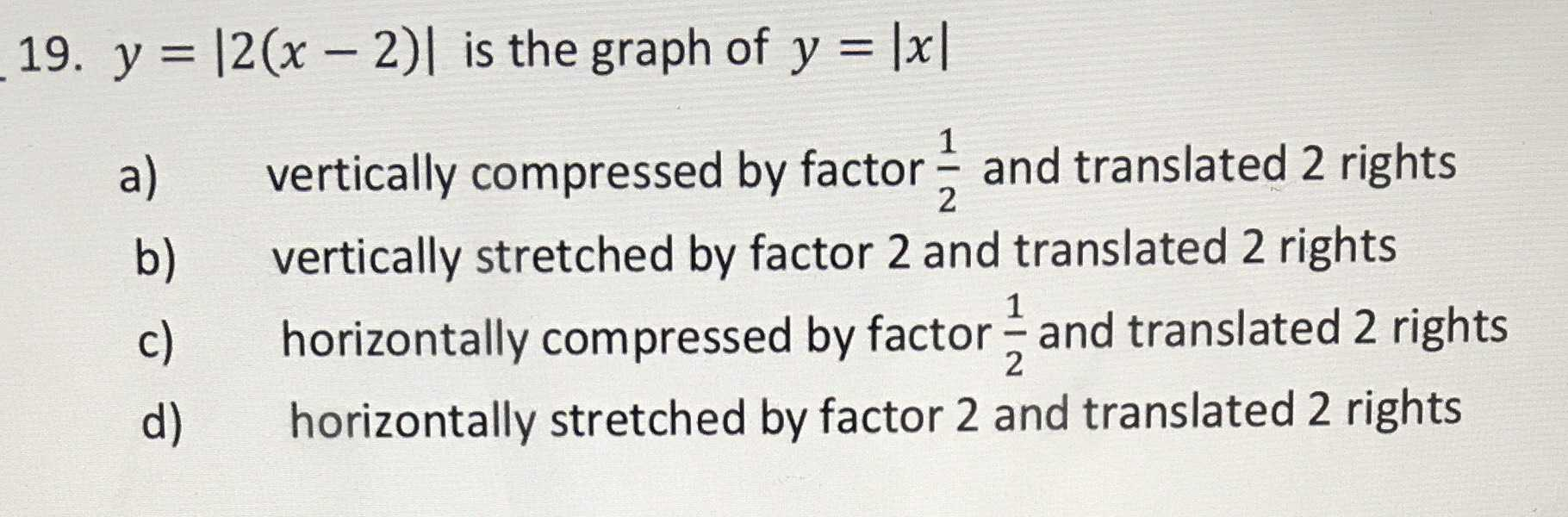### ¿Todavía tienes preguntas de matemáticas?

Pregunte a nuestros tutores expertos
Algebra
Pregunta19. $$y = | 2 ( x - 2 ) |$$ is the graph of $$y = | x |$$

a) vertically compressed by factor $$\frac { 1 } { 2 }$$ and translated $$2$$ rights

b) vertically stretched by factor $$2$$ and translated $$2$$ rights

c) horizontally compressed by factor $$\frac { 1 } { 2 }$$ and translated $$2$$ rights

d) horizontally stretched by factor $$2$$ and translated $$2$$ rights